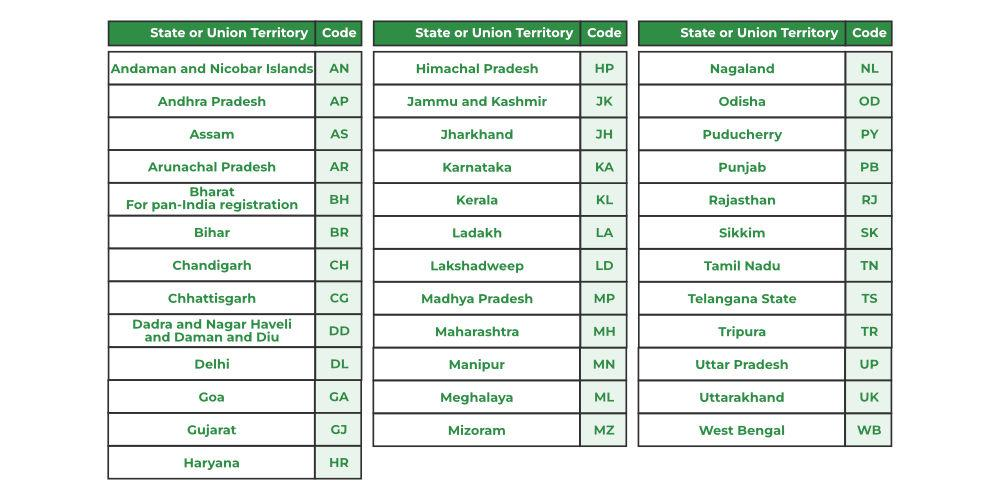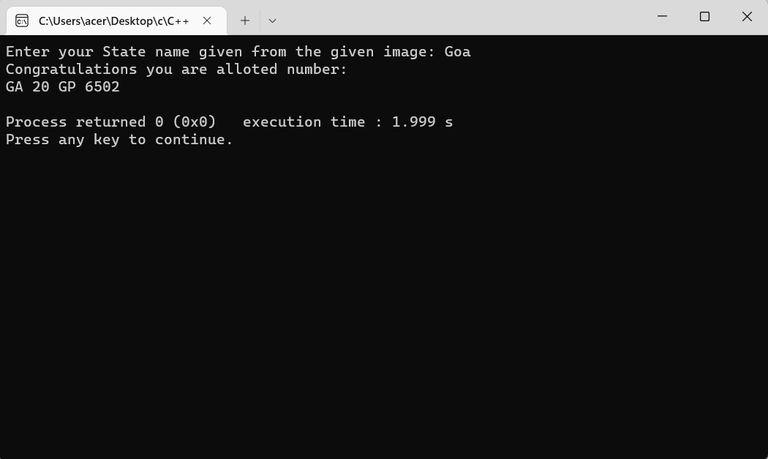Open in App
Not now

# Program to Generate Vehicle Number

• Last Updated : 13 Jan, 2023

Prerequisite:

In this article, we will be creating a simple java program to generate a unique number plate. for this let’s understand the format in which number plates can be generated.

The number plate for a vehicle consists of 4 parts:

1. State code : first 2 letters represent the State or Union Territory to which the vehicle is registered.
2. District code : a two-digit number allocated to a district within the respective state or Union Territory.
3. Single or Multiple Alphabet :  this represents unique alphabet for the number plate.
4. Number between 1 to 9999 :  it is assigned sequentially and unique for each registration.

Note:  we will consider this standard way for generating a number plate it can have certain variations based on country.

so let’s start implementation:

Step 1: we will create a list which will have list of all state .

Step 2: based on selected state we will generate a number under the range of available all district.

Step 3: here we will generate an unique alphabet and a number between 1 to 9999.

Step 4: here we will combine all things in a single variable to print it.

### States Code:Sheet showing data about states with their codes

let’s create a map that will have state names as keys and total available districts as values.

Elements to build number plate

state name+ district code(randomly generated according to state )+ unique generate alphabet+ random number

For Example:

Gujarat has 33 districts so the key would be GJ and the value would be 37(generates any number from 1 to 37)

Let’s breakdown the format to understanding.

Example: GJ 01 BC 0000

Example: We have used 1-37 random numbers as district numbers in the state but if you want to apply you need to make another nested map where we will store names of all the districts with the district code associated with it.

## C++

 `// C++ Program to implement` `// Number Plate Allocation` `#include ` `using` `namespace` `std;`   `void` `states_information(` `    ``unordered_map& states)` `{` `    ``states.insert({ ``"Andhra Pradesh"``, ``"AD"` `});` `    ``states.insert({ ``"Arunachal Pradesh"``, ``"AR"` `});` `    ``states.insert({ ``"Assam"``, ``"AS"` `});` `    ``states.insert({ ``"Bihar"``, ``"BR"` `});` `    ``states.insert({ ``"Chattisgarh"``, ``"CG"` `});` `    ``states.insert({ ``"Delhi"``, ``"DL"` `});` `    ``states.insert({ ``"Goa"``, ``"GA"` `});` `    ``states.insert({ ``"Gujarat"``, ``"GJ"` `});` `    ``states.insert({ ``"Haryana"``, ``"HR"` `});` `    ``states.insert({ ``"Himachal Pradesh"``, ``"HP"` `});` `    ``states.insert({ ``"Jammu and Kashmir"``, ``"JK"` `});` `    ``states.insert({ ``"Jharkhand"``, ``"JH"` `});` `    ``states.insert({ ``"Karnataka"``, ``"KA"` `});` `    ``states.insert({ ``"Kerala"``, ``"KL"` `});` `    ``states.insert({ ``"Lakshadweep Islands"``, ``"LD"` `});` `    ``states.insert({ ``"Madhya Pradesh"``, ``"MP"` `});` `    ``states.insert({ ``"Maharashtra"``, ``"MH"` `});` `    ``states.insert({ ``"Manipur"``, ``"MN"` `});` `    ``states.insert({ ``"Meghalaya"``, ``"ML"` `});` `    ``states.insert({ ``"Mizoram"``, ``"MZ"` `});` `    ``states.insert({ ``"Nagaland"``, ``"NL"` `});` `    ``states.insert({ ``"Odisha"``, ``"OD"` `});` `    ``states.insert({ ``"Pondicherry"``, ``"PY"` `});` `    ``states.insert({ ``"Punjab"``, ``"PB"` `});` `    ``states.insert({ ``"Rajasthan"``, ``"RJ"` `});` `    ``states.insert({ ``"Sikkim"``, ``"SK"` `});` `    ``states.insert({ ``"Tamil Nadu"``, ``"TN"` `});` `    ``states.insert({ ``"Telangana"``, ``"TS"` `});` `    ``states.insert({ ``"Tripura"``, ``"TR"` `});` `    ``states.insert({ ``"Uttar Pradesh"``, ``"UP"` `});` `    ``states.insert({ ``"Uttarakhand"``, ``"UK"` `});` `    ``states.insert({ ``"West Bengal"``, ``"WB"` `});` `    ``states.insert({ ``"Andaman and Nicobar Islands"``, ``"AN"` `});` `    ``states.insert({ ``"Chandigarh"``, ``"CH"` `});` `    ``states.insert({ ``"Dadra & Nagar Haveli and Daman & Diu"``,` `                    ``"DNHDD"` `});` `    ``states.insert({ ``"Ladakh"``, ``"LA"` `});` `}`   `int` `main()` `{` `    ``// Declaring Map to store states and state codes` `    ``unordered_map states;`   `    ``// Calling function to update data` `    ``states_information(states);`   `    ``string number_alloted = ``""``;`   `    ``// Input the state name` `    ``string place;` `    ``cout << ``"Enter your State name given from the given "` `            ``"image: "``;` `    ``cin >> place;`   `    ``string temp = to_string((``rand``() % 31) + 10);` `    ``char` `char1 = (``rand``() % 26) + 64;` `    ``char` `char2 = (``rand``() % 26) + 64;`   `    ``int` `last_digits = ``rand``() % 9999;`   `    ``cout << ``"Congratulations you are alloted number:\n"``;`   `    ``// Number allocated is calculated` `    ``number_alloted = states[place] + ``" "` `+ temp + ``" "` `                     ``+ char1 + char2 + ``" "` `                     ``+ to_string(last_digits);` `    ``cout << number_alloted << endl;`   `    ``return` `0;` `}`

## Java

 `// Java Program to implement` `// Number Plate Allocation` `import` `java.util.*;`   `public` `class` `Main {` `    ``public` `static` `HashMap state_code;`   `    ``// Function to update states and state_code` `    ``public` `static` `void` `    ``state_data(HashMap states)` `    ``{` `        ``states.put(``"Andhra Pradesh"``, ``"AD"``);` `        ``states.put(``"Arunachal Pradesh"``, ``"AR"``);` `        ``states.put(``"Assam"``, ``"AS"``);` `        ``states.put(``"Bihar"``, ``"BR"``);` `        ``states.put(``"Chattisgarh"``, ``"CG"``);` `        ``states.put(``"Delhi"``, ``"DL"``);` `        ``states.put(``"Goa"``, ``"GA"``);` `        ``states.put(``"Gujarat"``, ``"GJ"``);` `        ``states.put(``"Haryana"``, ``"HR"``);` `        ``states.put(``"Himachal Pradesh"``, ``"HP"``);` `        ``states.put(``"Jammu and Kashmir"``, ``"JK"``);` `        ``states.put(``"Jharkhand"``, ``"JH"``);` `        ``states.put(``"Karnataka"``, ``"KA"``);` `        ``states.put(``"Kerala"``, ``"KL"``);` `        ``states.put(``"Lakshadweep Islands"``, ``"LD"``);` `        ``states.put(``"Madhya Pradesh"``, ``"MP"``);` `        ``states.put(``"Maharashtra"``, ``"MH"``);` `        ``states.put(``"Manipur"``, ``"MN"``);` `        ``states.put(``"Meghalaya"``, ``"ML"``);` `        ``states.put(``"Mizoram"``, ``"MZ"``);` `        ``states.put(``"Nagaland"``, ``"NL"``);` `        ``states.put(``"Odisha"``, ``"OD"``);` `        ``states.put(``"Pondicherry"``, ``"PY"``);` `        ``states.put(``"Punjab"``, ``"PB"``);` `        ``states.put(``"Rajasthan"``, ``"RJ"``);` `        ``states.put(``"Sikkim"``, ``"SK"``);` `        ``states.put(``"Tamil Nadu"``, ``"TN"``);` `        ``states.put(``"Telangana"``, ``"TS"``);` `        ``states.put(``"Tripura"``, ``"TR"``);` `        ``states.put(``"Uttar Pradesh"``, ``"UP"``);` `        ``states.put(``"Uttarakhand"``, ``"UK"``);` `        ``states.put(``"West Bengal"``, ``"WB"``);` `        ``states.put(``"Andaman and Nicobar Islands"``, ``"AN"``);` `        ``states.put(``"Chandigarh"``, ``"CH"``);` `        ``states.put(``"Dadra & Nagar Haveli and Daman & Diu"``,` `                   ``"DNHDD"``);` `        ``states.put(``"Ladakh"``, ``"LA"``);` `    ``}`   `    ``public` `static` `void` `main(String[] args)` `    ``{`   `        ``HashMap states = ``new` `HashMap<>();` `        ``Random rand = ``new` `Random();`   `        ``Scanner scn = ``new` `Scanner(System.in);`   `        ``state_data(states);`   `        ``int` `no_of_states = states.size();`   `        ``// accesing random index from arrayList` `        ``int` `random_index = rand.nextInt(no_of_states);`   `        ``String number = String.valueOf(` `            ``rand.nextInt(``9999``)); ``// 0 to 9999`   `        ``System.out.println(` `            ``"Enter your State name given from the given image: "``);` `        ``String str2 = scn.nextLine();`   `        ``String selected_State = states.get(str2);`   `        ``// now based on selected state we map it with` `        ``// available total number of district with Hashmap` `        ``// generating a city` `        ``// number under the range` `        ``// of available total city` `        ``// for selected state` `        ``String selected_District_Code` `            ``= String.valueOf(rand.nextInt(``37``));`   `        ``// if district code is  less than 9 then add 0 in` `        ``// prefix` `        ``selected_District_Code` `            ``= (Integer.parseInt(selected_District_Code)` `               ``< ``10``)` `                  ``? ``"0"` `+ selected_District_Code` `                  ``: selected_District_Code;`   `        ``// now we will generate  2 unique alphabets` `        ``char` `c = (``char``)(``'A'` `+ rand.nextInt(``26``));` `        ``char` `c2 = (``char``)(``'A'` `+ rand.nextInt(``26``));`   `        ``// Let's combine all the things` `        ``String vehicle_NumberPlate` `            ``= selected_State + ``" "` `+ selected_District_Code` `              ``+ ``" "` `+ c + c2 + ``" "` `+ number;`   `        ``System.out.println(` `            ``"Congratulations you are alloted number:"``);` `        ``System.out.println(vehicle_NumberPlate);` `    ``}` `}`

Output:The output of the Program

My Personal Notes arrow_drop_up
Related Articles